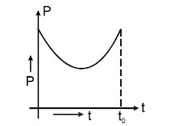Power
Question

# Power versus time graph for a given force is given below. Work done by the force upto time $\mathrm{t}\left(\le {\mathrm{t}}_{0}\right)$ .Easy
Solution

## Area of P-t graph is work done. As with t increases area under the graph increases so work done also increases

Get Instant Solutions